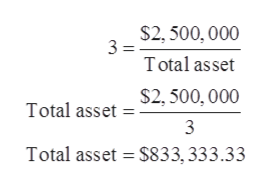# Cream of Tomato Company has the following data available.Net income = \$250,000Sales = \$2.5 millionTotal asset turnover = 3Equity multiplier = 2.5Cream of Tomato does not have any preferred stock outstanding. Note that the equitymultiplier is defined as total assets to equity. Calculate the return on assets and return onequity for Cream of Tomato.

Question
1 views

Cream of Tomato Company has the following data available.
Net income = \$250,000
Sales = \$2.5 million
Total asset turnover = 3
Equity multiplier = 2.5
Cream of Tomato does not have any preferred stock outstanding. Note that the equity
multiplier is defined as total assets to equity. Calculate the return on assets and return on
equity for Cream of Tomato.

check_circle

Step 1

The formula for total asset turnover is given below:

Step 2

Substitute 3 for total asset turnover ratio and \$2,500,000 for net sales,help_outlineImage Transcriptionclose\$2, 500, 000 3 = Total asset \$2, 500, 000 Total asset 3 Total asset = \$833, 333.33 fullscreen
Step 3

Equity multiplier is 2.5.

The formula for e...

### Want to see the full answer?

See Solution

#### Want to see this answer and more?

Solutions are written by subject experts who are available 24/7. Questions are typically answered within 1 hour.*

See Solution
*Response times may vary by subject and question.
Tagged in